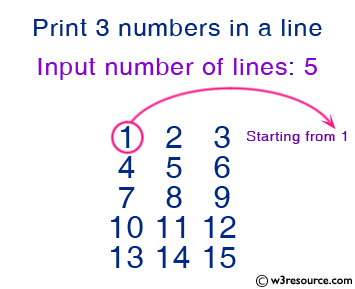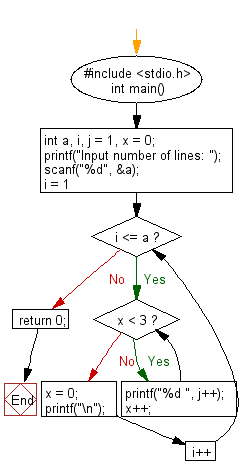﻿ C : Print 3 numbers in a line, starting from 1 to n lines

# C Exercises: Print 3 numbers in a line, starting from 1 and print n lines

## C Basic Declarations and Expressions: Exercise-41 with Solution

Write a C program to print 3 numbers on a line, starting with 1 and printing n lines. Accept the number of lines (n, integer) from the user.

Pictorial Presentation:Sample Solution:

C Code:

``````#include <stdio.h>
int main() {
int a, i, j = 1, x = 0;

// Prompt for user input
printf("Input number of lines: ");
scanf("%d", &a);

// Loop for each line
for(i = 1; i <= a; i++) {
// Loop to print numbers
while(x < 3) {
printf("%d ", j++);
x++;
}
x = 0; // Reset the counter
printf("\n");
}

return 0;
}
``````

Sample Output:

```Input number of lines: 5
1 2 3
4 5 6
7 8 9
10 11 12
13 14 15
```

Flowchart:C programming Code Editor:

What is the difficulty level of this exercise?

Test your Programming skills with w3resource's quiz.

﻿